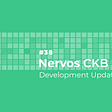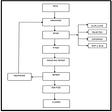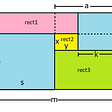LeetCode: 561. Array Partition I

This problem published on the LeetCode, is one that deals with the concepts of array.Let’s have a look in to the problem description.

Given an integer array `nums` of `2n` integers, group these integers into `n` pairs `(a1, b1), (a2, b2), ..., (an, bn)` such that the sum of `min(ai, bi)` for all `i` is maximized. Return the maximized sum

Example 1

Input: nums = [1,4,3,2]
Output: 4
Explanation: All possible pairings (ignoring the ordering of elements) are:
1. (1, 4), (2, 3) -> min(1, 4) + min(2, 3) = 1 + 2 = 3
2. (1, 3), (2, 4) -> min(1, 3) + min(2, 4) = 1 + 2 = 3
3. (1, 2), (3, 4) -> min(1, 2) + min(3, 4) = 1 + 3 = 4
So the maximum possible sum is 4.

Example 2

Input: nums = [6,2,6,5,1,2]
Output: 9
Explanation: The optimal pairing is (2, 1), (2, 5), (6, 6). min(2, 1) + min(2, 5) + min(6, 6) = 1 + 2 + 6 = 9.

The solution that I would basically gonna describe is of the order of ‘n’ time complexity. If we carefully read the problem, we find that , the problem simply aims at pairing all the numbers in the array such that, sum of min(a,b) is maximum. So on careful understanding , we find, this is possible just by using any of the o(nlgn) sorting, and then making the pairs of 2 adjacent numbers. This would give the sum to the maximum value.

The link for the solution is given below:

https://leetcode.com/problems/array-partition-i/

--

--

--

## More from Dhanarajappu

Tech-Enthusiast, Coder,Explorer,Geeky,Software Engineer |A piece of code delivers everything that you need. The world is all about codes.

Love podcasts or audiobooks? Learn on the go with our new app.

## Day 88: Moving Platforms in Unity## Printing and scanning from a distance with Raspberry Pi and your old USB printer.## Nervos CKB Development Update #38## Practical Defect/Bug Life Cycle followed in IT companies## Laravel Breeze Tutorial: The Definitive Guide (2021)## Dhanarajappu

Tech-Enthusiast, Coder,Explorer,Geeky,Software Engineer |A piece of code delivers everything that you need. The world is all about codes.

## Notes from LeetCode## CS373 Spring 2022: Elliot Sims## CS373 Spring 2022: Nathan Whyte